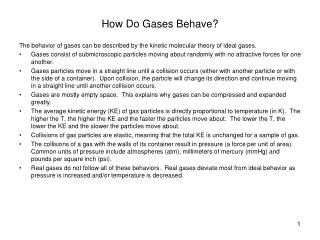DownloadDownload PresentationHow Do Gases Behave?

# How Do Gases Behave?

Download Presentation## How Do Gases Behave?

- - - - - - - - - - - - - - - - - - - - - - - - - - - E N D - - - - - - - - - - - - - - - - - - - - - - - - - - -
##### Presentation Transcript

1. How Do Gases Behave? The behavior of gases can be described by the kinetic molecular theory of ideal gases. • Gases consist of submicroscopic particles moving about randomly with no attractive forces for one another. • Gases particles move in a straight line until a collision occurs (either with another particle or with the side of a container). Upon collision, the particle will change its direction and continue moving in a straight line until another collision occurs. • Gases are mostly empty space. This explains why gases can be compressed and expanded greatly. • The average kinetic energy (KE) of gas particles is directly proportional to temperature (in K). The higher the T, the higher the KE and the faster the particles move about. The lower the T, the lower the KE and the slower the particles move about. • Collisions of gas particles are elastic, meaning that the total KE is unchanged for a sample of gas. • The collisions of a gas with the walls of its container result in pressure (a force per unit of area). Common units of pressure include atmospheres (atm), millimeters of mercury (mmHg) and pounds per square inch (psi). • Real gases do not follow all of these behaviors. Real gases deviate most from ideal behavior as pressure is increased and/or temperature is decreased.

2. How Do Gases Behave? • Boyle’s Law: the volume of a fixed amount of gas at constant temperature is inversely proportional to its pressure. • Charles’s Law: the volume of a fixed amount of gas at constant pressure is directly proportional to its Kelvin temperature. • Gay-Lussac’s Law: the pressure of a fixed amount of gas at a constant volume is directly proportional to its Kelvin temerature. • Avogadro’s Law: the volume of a gas is directly proportional to its molar amount at a constant pressure and temperature; one mole of a gas at STP is always 22.4 L (the molar volume of all gases).

3. How Do Gases Behave?

4. Ch 8 Problems-Gases • How does the volume of a gas change if the temperature is increased while the pressure and moles are held constant? • How does the volume of a gas change if the pressure is increased while the temperature and number of moles are held constant? • Convert 0.820 atm into mmHg. HINT: 760 mmHg = 1 atm • A balloon indoors at a temperature of 27°C has a volume of 2.00 L. What is the new volume if the balloon is taken outside where the temperature is -23°C? Assume no change in pressure and moles. • What volume is occupied by 4.11 g of methane gas (CH4) at STP?